Examples

Chapter 10 Class 10 Circles
Serial order wise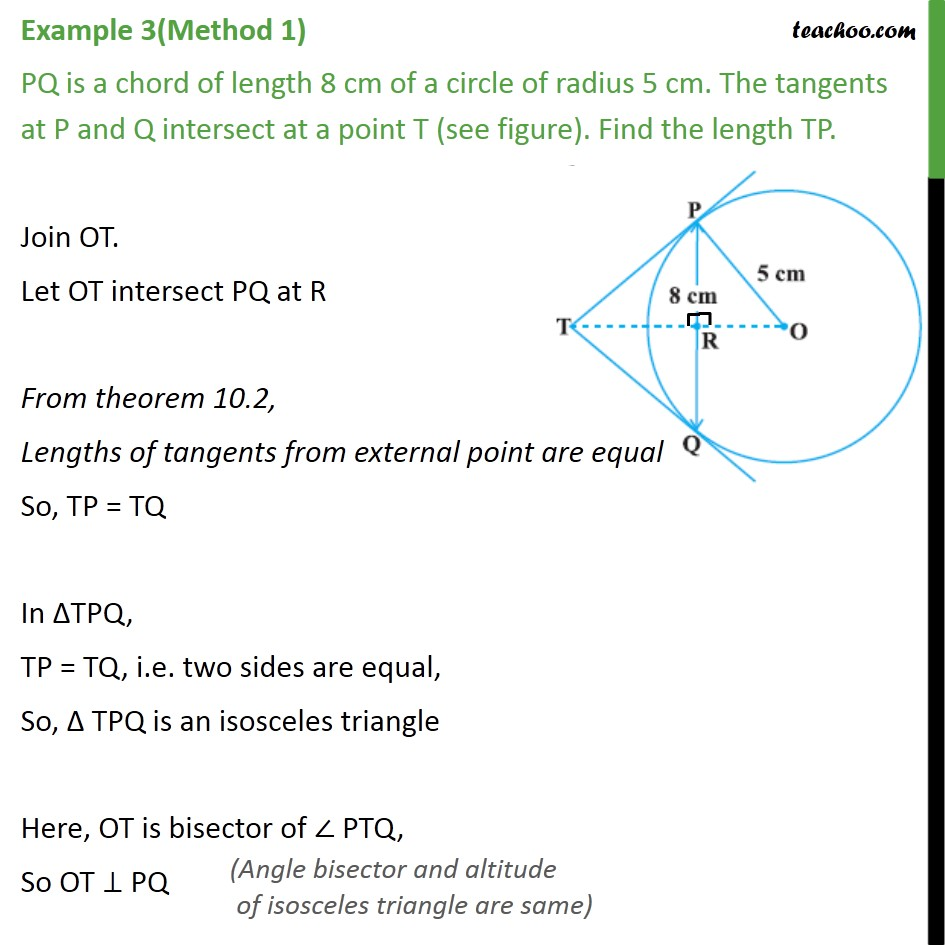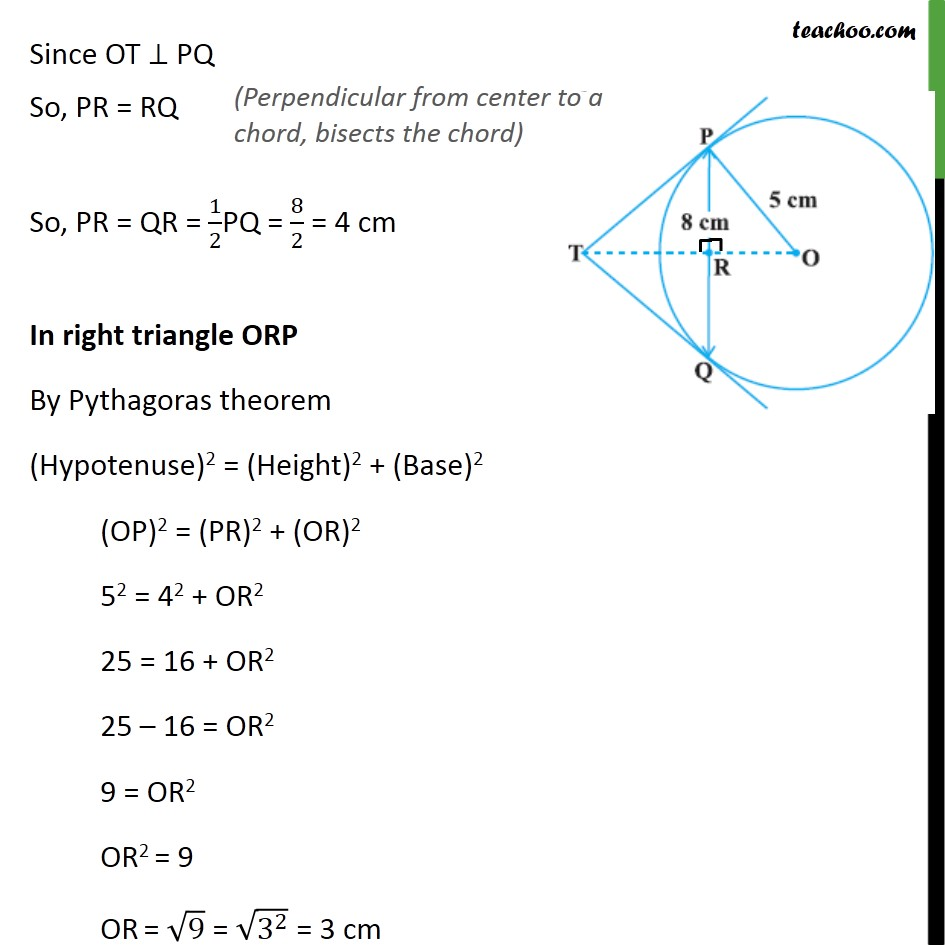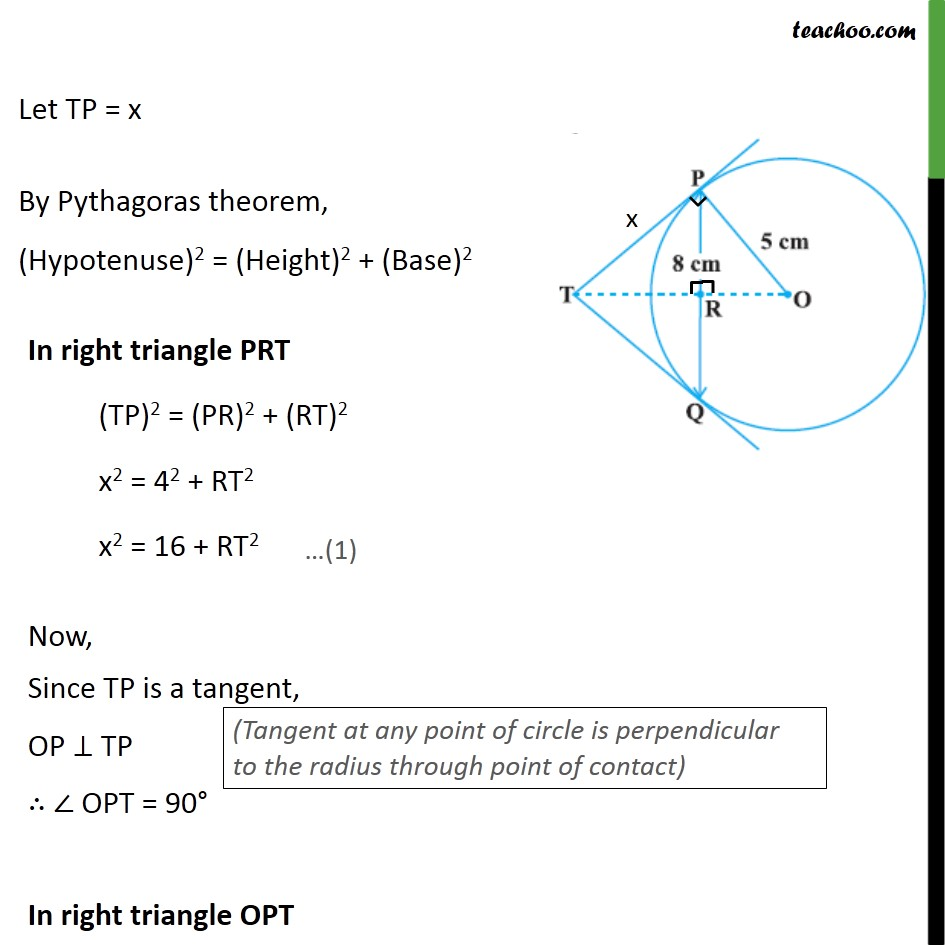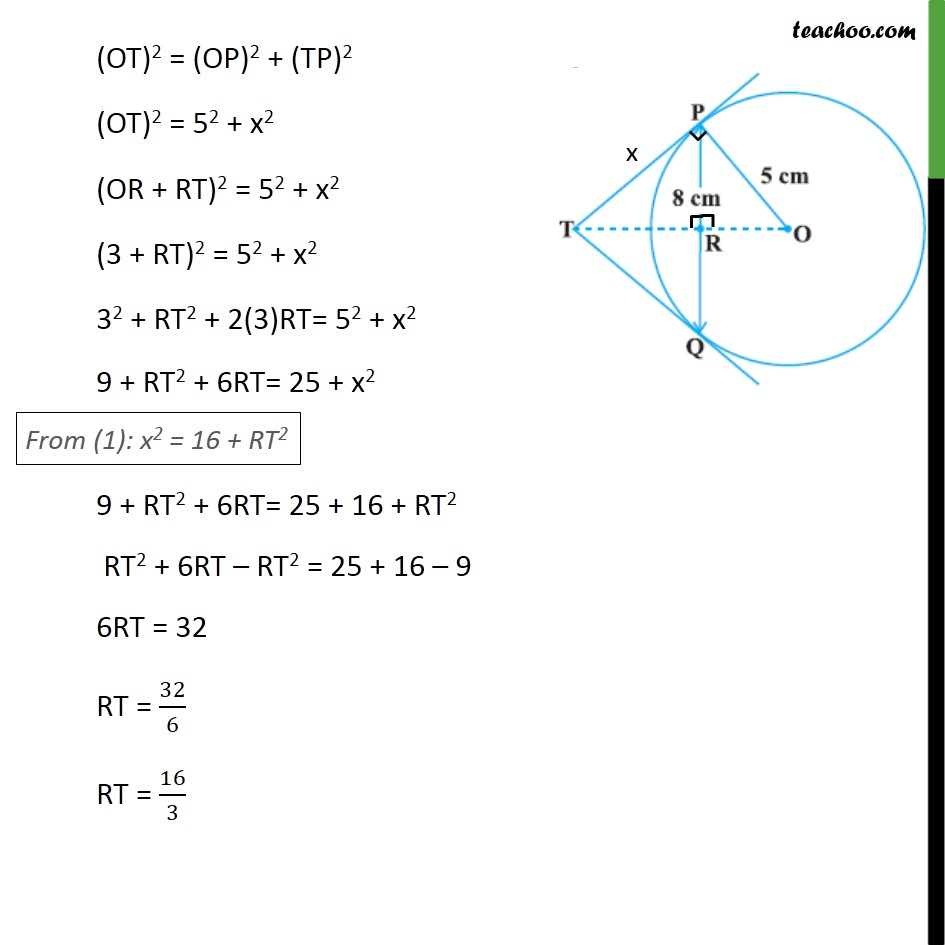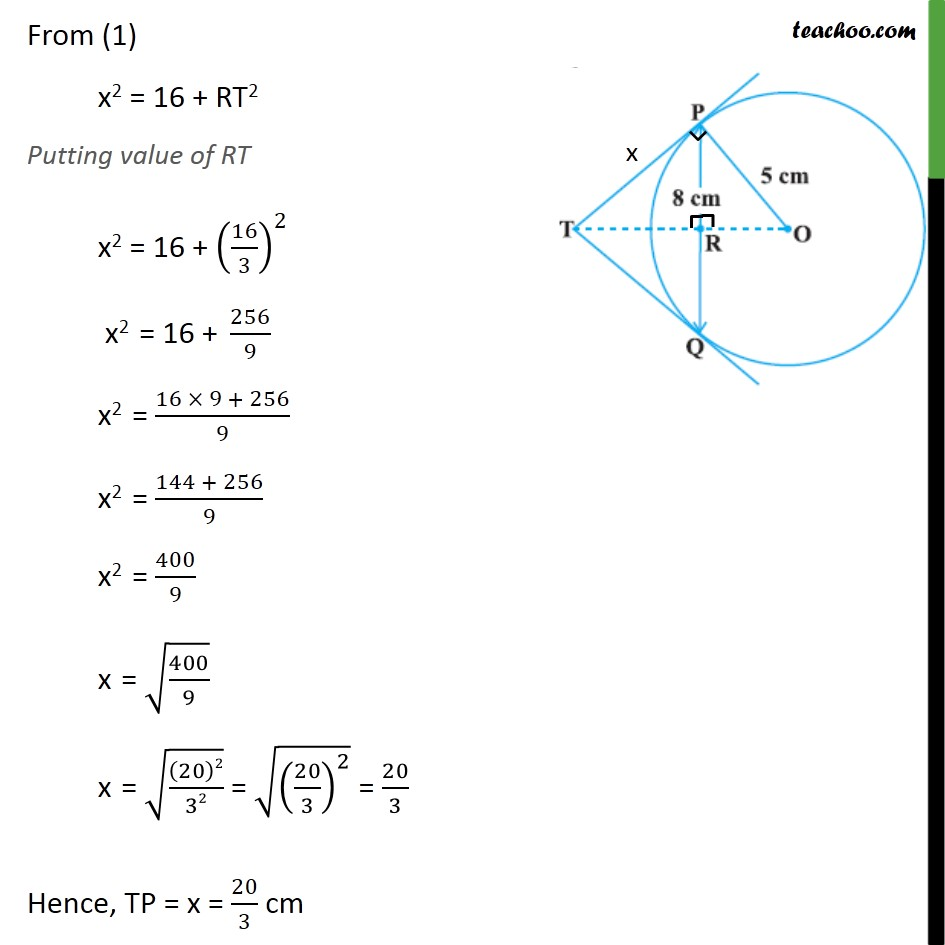This video is only available for Teachoo black users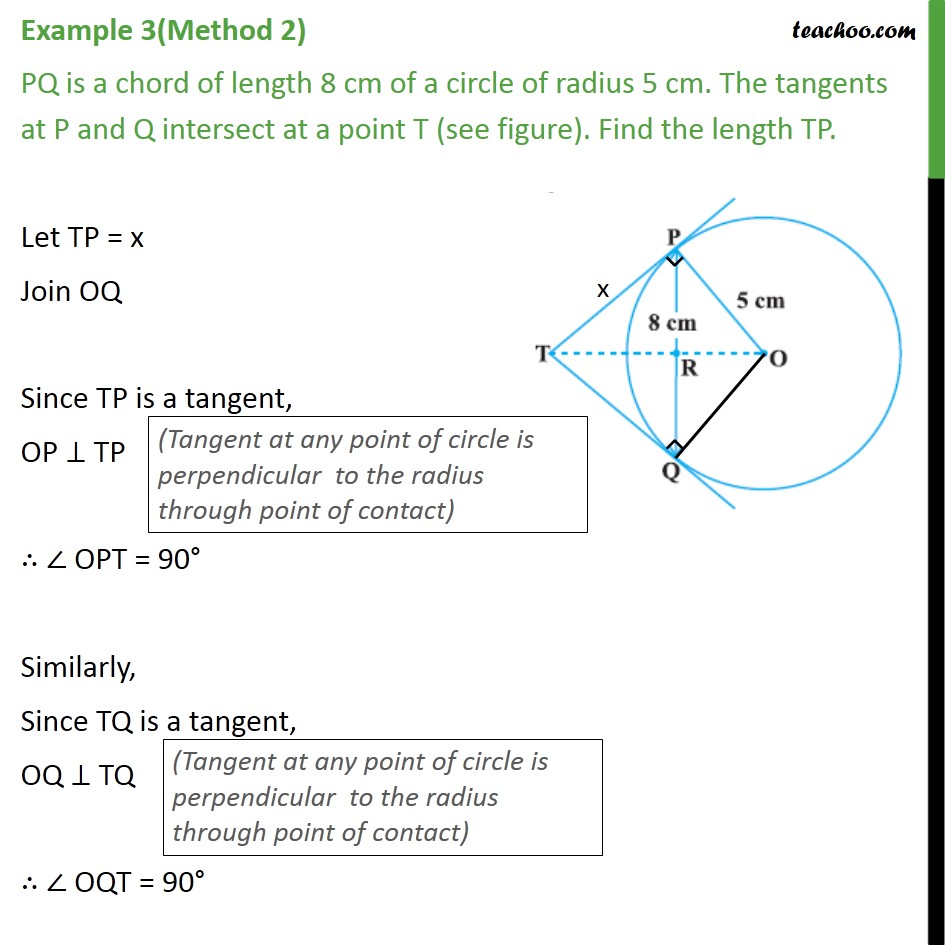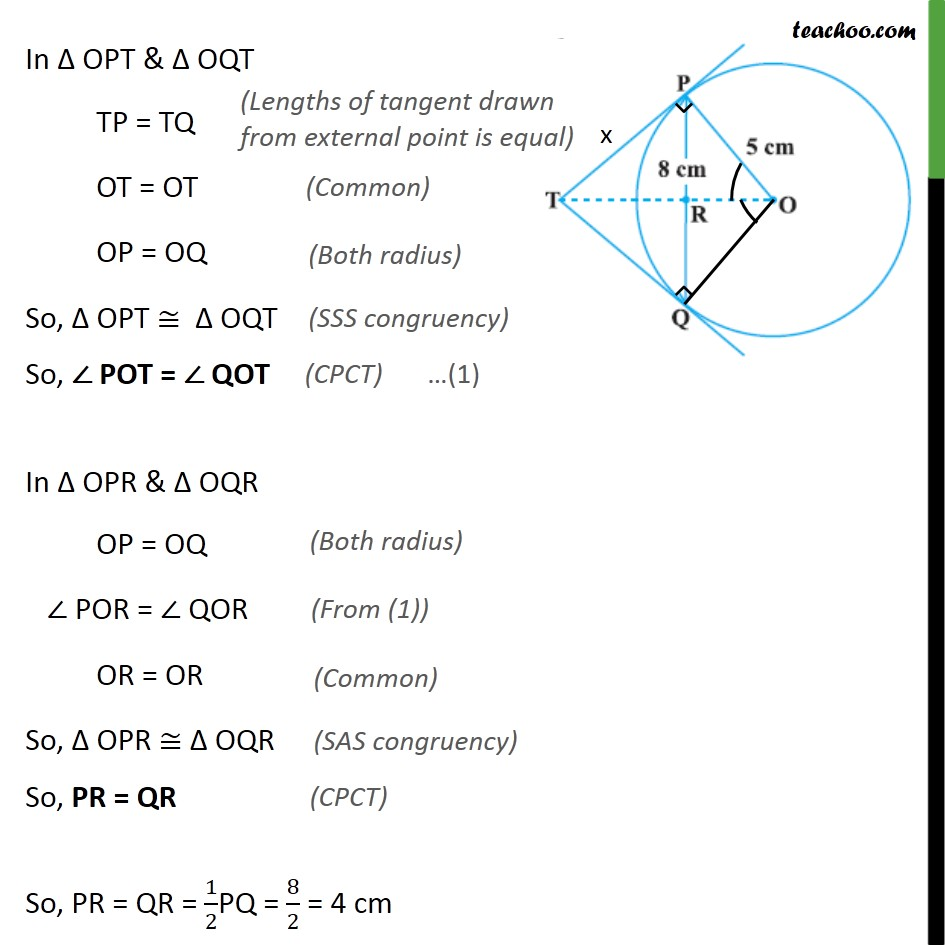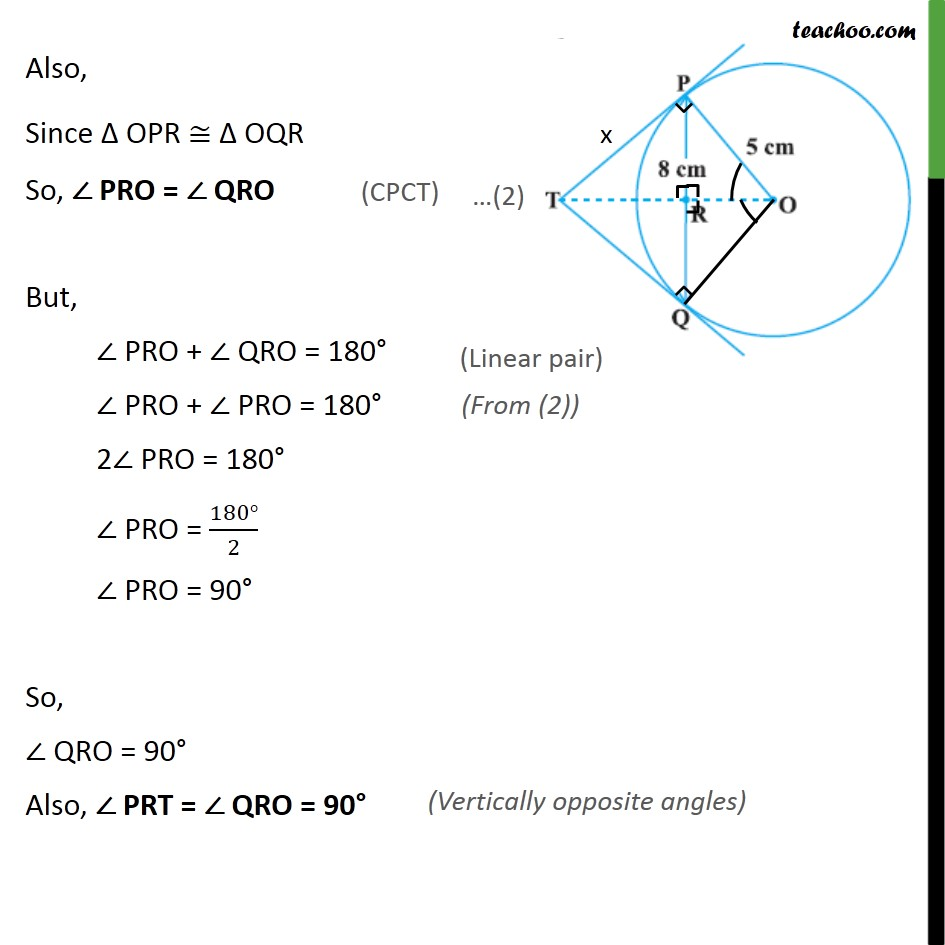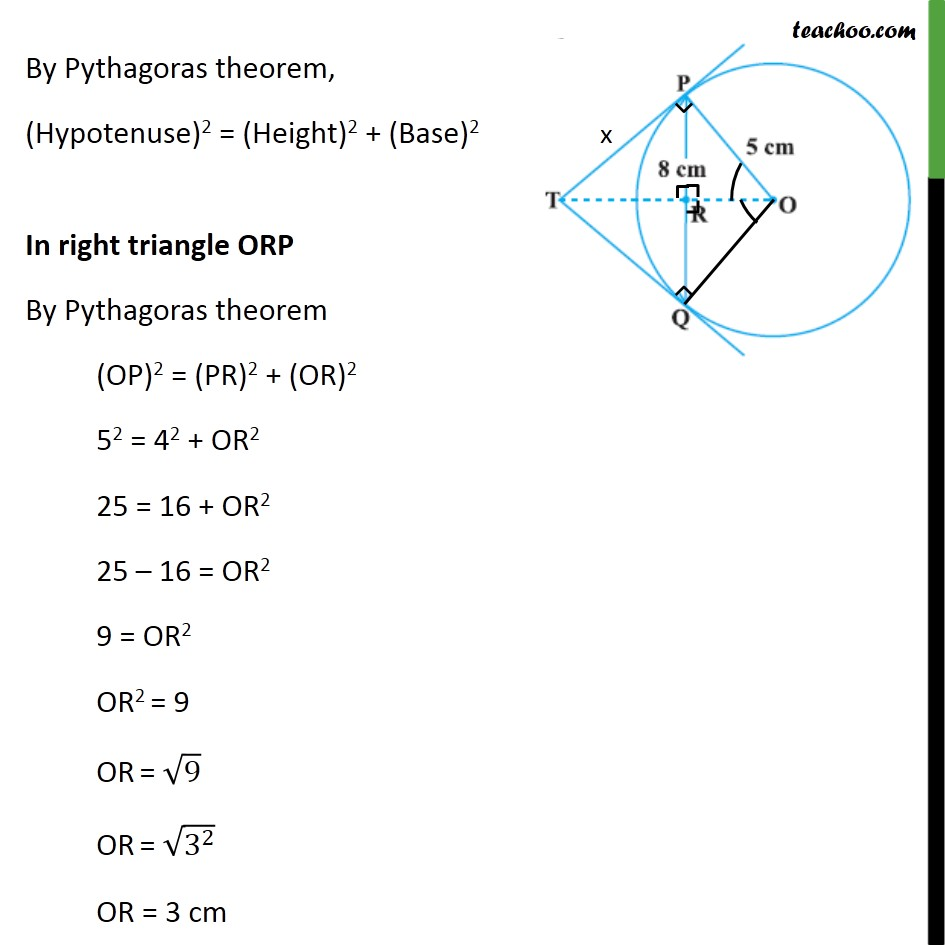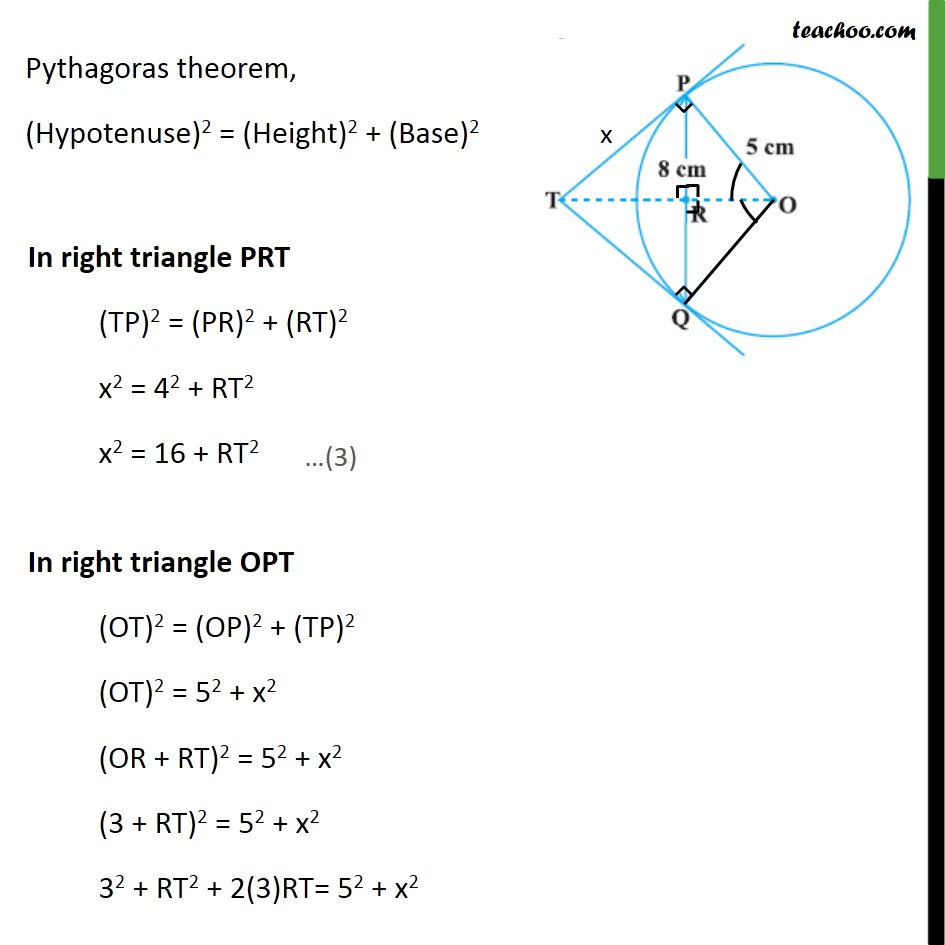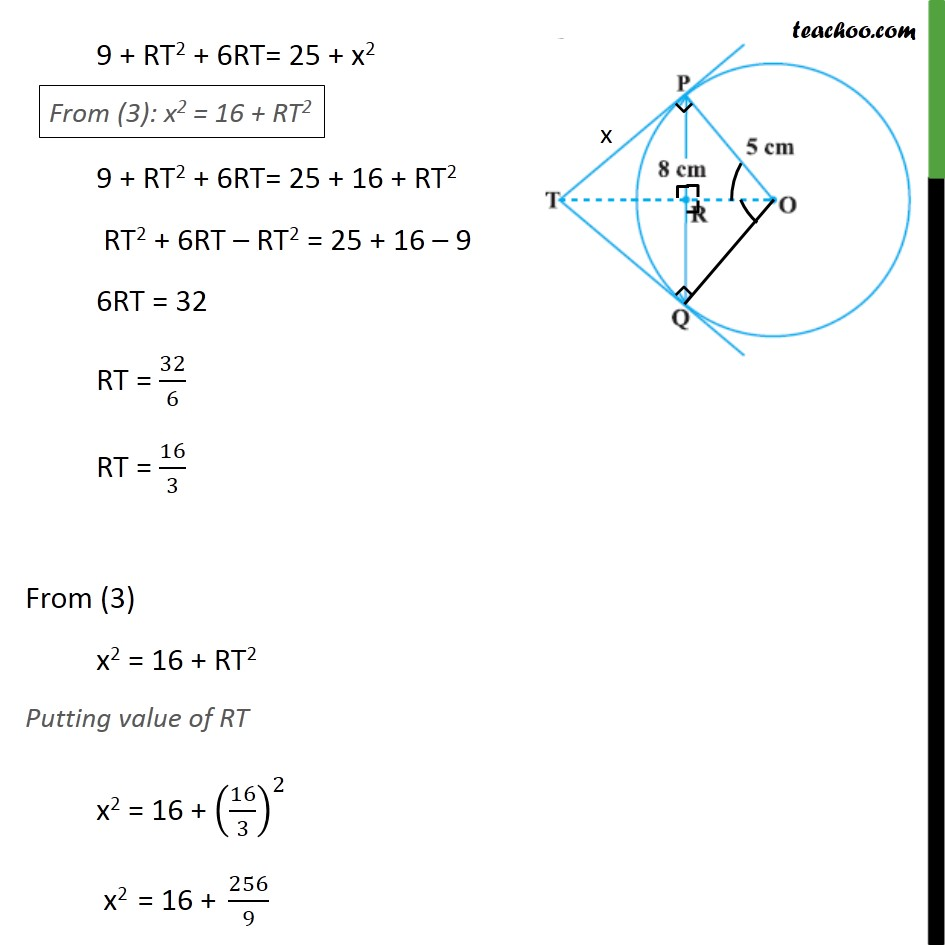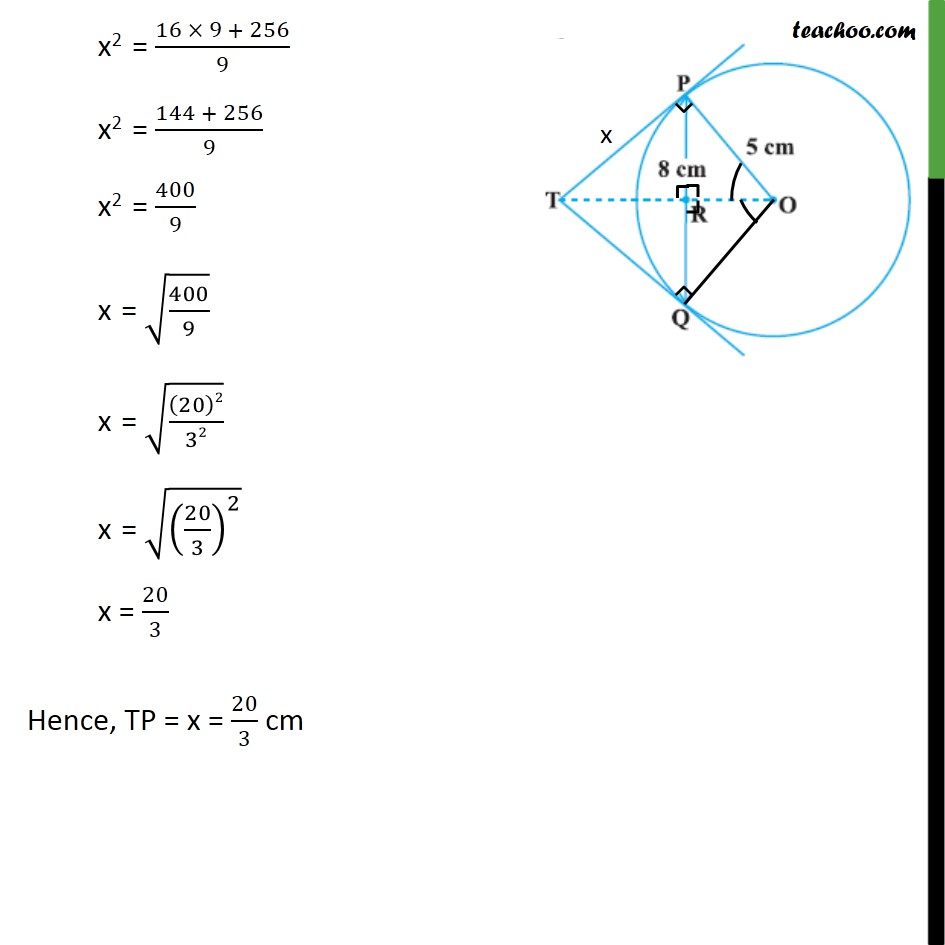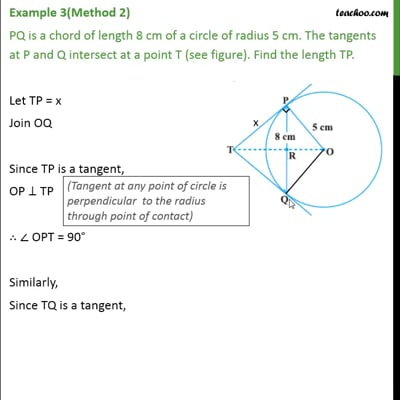This video is only available for Teachoo black users

Solve all your doubts with Teachoo Black (new monthly pack available now!)

### Transcript

Example 3(Method 1) PQ is a chord of length 8 cm of a circle of radius 5 cm. The tangents at P and Q intersect at a point T (see figure). Find the length TP. Join OT. Let OT intersect PQ at R From theorem 10.2, Lengths of tangents from external point are equal So, TP = TQ In ΔTPQ, TP = TQ, i.e. two sides are equal, So, Δ TPQ is an isosceles triangle Here, OT is bisector of ∠ PTQ, So OT ⊥ PQ Since OT ⊥ PQ So, PR = RQ So, PR = QR = 1/2PQ = 8/2 = 4 cm In right triangle ORP By Pythagoras theorem (Hypotenuse)2 = (Height)2 + (Base)2 (OP)2 = (PR)2 + (OR)2 52 = 42 + OR2 25 = 16 + OR2 25 – 16 = OR2 9 = OR2 OR2 = 9 OR = √9 = √(3^2 ) = 3 cm Let TP = x By Pythagoras theorem, (Hypotenuse)2 = (Height)2 + (Base)2 (OT)2 = (OP)2 + (TP)2 (OT)2 = 52 + x2 (OR + RT)2 = 52 + x2 (3 + RT)2 = 52 + x2 32 + RT2 + 2(3)RT= 52 + x2 9 + RT2 + 6RT= 25 + x2 9 + RT2 + 6RT= 25 + 16 + RT2 RT2 + 6RT – RT2 = 25 + 16 – 9 6RT = 32 RT = 32/6 RT = 16/3 From (1) x2 = 16 + RT2 Putting value of RT x2 = 16 + (16/3)^2 x2 = 16 + 256/9 x2 = (16 × 9 + 256)/9 x2 = (144 + 256)/9 x2 = 400/9 x = √(400/9) x = √((20)2/32) = √((20/3)^2 ) = 20/3 Hence, TP = x = 20/3 cm Example 3(Method 2) PQ is a chord of length 8 cm of a circle of radius 5 cm. The tangents at P and Q intersect at a point T (see figure). Find the length TP. Let TP = x Join OQ Since TP is a tangent, OP ⊥ TP ∴ ∠ OPT = 90° Similarly, Since TQ is a tangent, OQ ⊥ TQ ∴ ∠ OQT = 90° In Δ OPT & Δ OQT TP = TQ OT = OT OP = OQ So, Δ OPT ≅ Δ OQT So, ∠ POT = ∠ QOT In Δ OPR & Δ OQR OP = OQ ∠ POR = ∠ QOR OR = OR So, Δ OPR ≅ Δ OQR So, PR = QR So, PR = QR = 1/2PQ = 8/2 = 4 cm Also, Since Δ OPR ≅ Δ OQR So, ∠ PRO = ∠ QRO But, ∠ PRO + ∠ QRO = 180° ∠ PRO + ∠ PRO = 180° 2∠ PRO = 180° ∠ PRO = (180°)/2 ∠ PRO = 90° So, ∠ QRO = 90° Also, ∠ PRT = ∠ QRO = 90° By Pythagoras theorem, (Hypotenuse)2 = (Height)2 + (Base)2 In right triangle ORP By Pythagoras theorem (OP)2 = (PR)2 + (OR)2 52 = 42 + OR2 25 = 16 + OR2 25 – 16 = OR2 9 = OR2 OR2 = 9 OR = √9 OR = √(3^2 ) OR = 3 cm Pythagoras theorem, (Hypotenuse)2 = (Height)2 + (Base)2 9 + RT2 + 6RT= 25 + x2 9 + RT2 + 6RT= 25 + 16 + RT2 RT2 + 6RT – RT2 = 25 + 16 – 9 6RT = 32 RT = 32/6 RT = 16/3 From (3) x2 = 16 + RT2 Putting value of RT x2 = 16 + (16/3)^2 x2 = 16 + 256/9 x2 = (16 × 9 + 256)/9 x2 = (144 + 256)/9 x2 = 400/9 x = √(400/9) x = √((20)2/32) x = √((20/3)^2 ) x = 20/3 Hence, TP = x = 20/3 cm